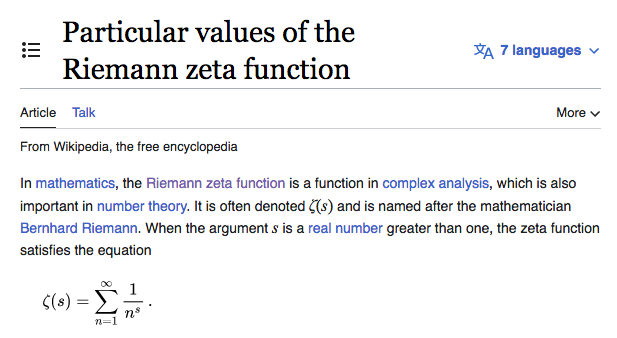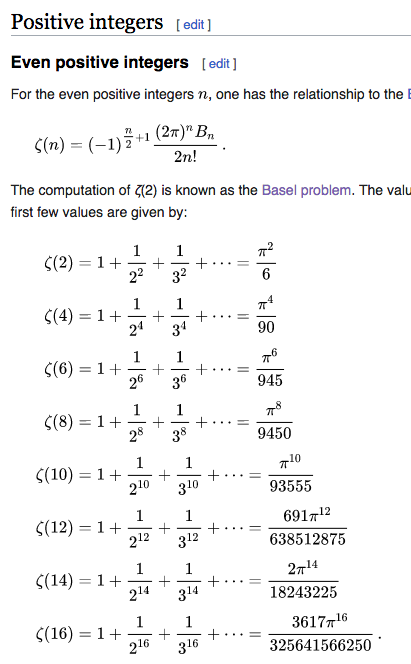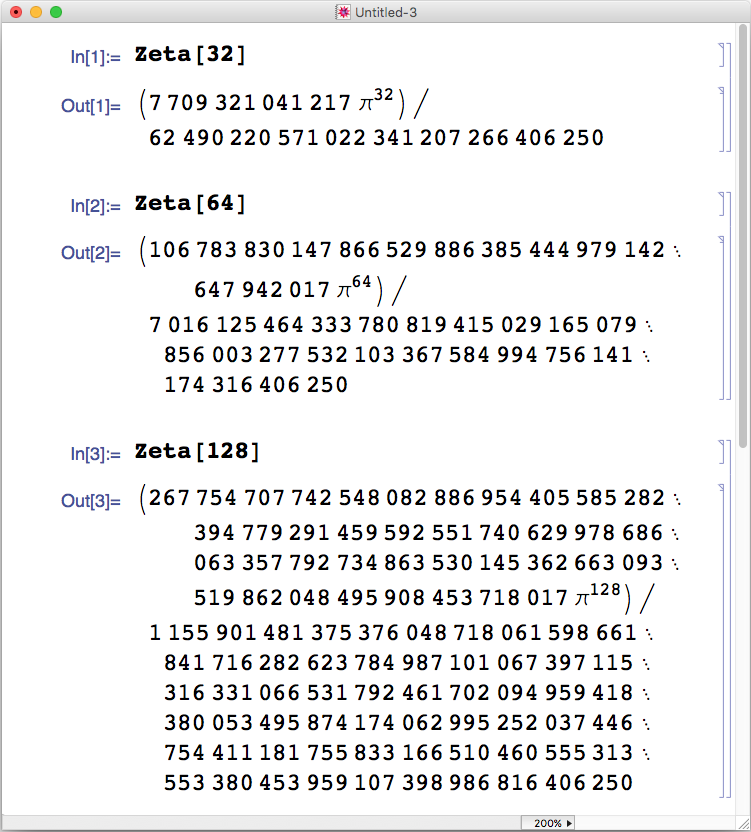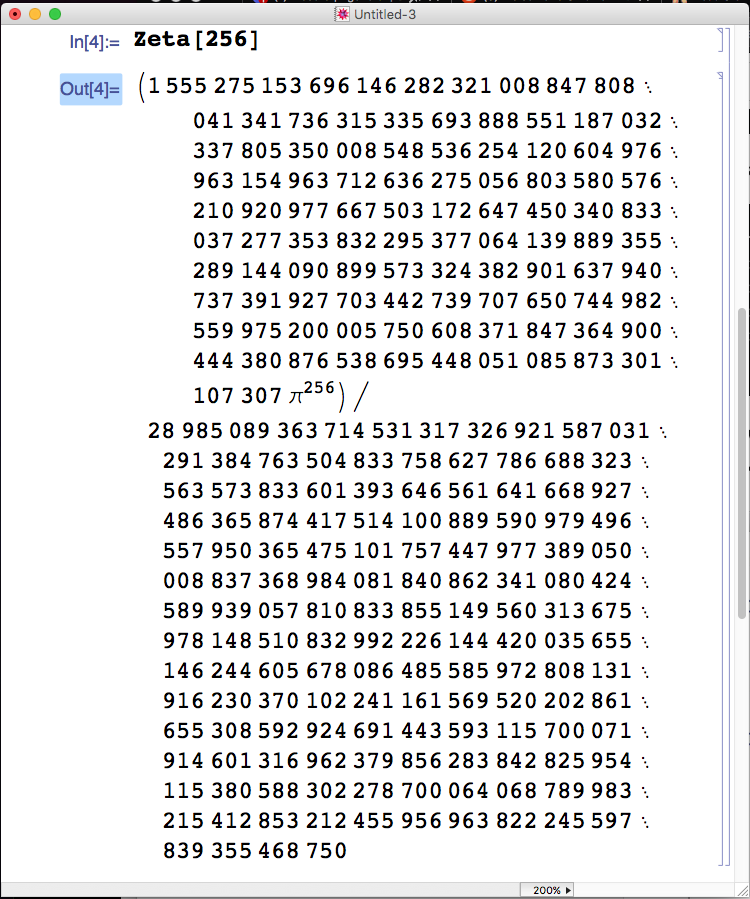## Julia Community 🟣

Steven Siew

Posted on • Updated on

# Using Riemann Zeta Function and DoubleFloats to calculate Pi

## Riemann Zeta FunctionIt has the following particular values## DoubleFloats

DoubleFloats uses two Float64 instances to represent a single floating point number with 85 significant bits.

In this article we use it to calculate Pi because it can calculate pi to about 30 decimal digits where as normal Float64 only have 15 decimal digits.

## Program

``````using DoubleFloats

function calc_zeta_02(n)
local accumulator = Double64(0)
for k = n:-1:1
accumulator += Double64(1)/(Double64(k)^2)
end
result = Double64(6) * accumulator
result = sqrt(result)
return result
end

function calc_zeta_04(n)
local accumulator = Double64(0)
for k = n:-1:1
accumulator += Double64(1)/(Double64(k)^4)
end
result = Double64(90) * accumulator
result = sqrt(sqrt(result))
return result
end

function calc_zeta_08(n)
local accumulator = Double64(0)
for k = n:-1:1
accumulator += Double64(1)/(Double64(k)^8)
end
result = Double64(9450) * accumulator
result = sqrt(sqrt(sqrt(result)))
return result
end

function calc_zeta_16(n)
local accumulator = Double64(0)
for k = n:-1:1
accumulator += Double64(1)/(Double64(k)^16)
end
result = Double64("325641566250") / Double64(3617) * accumulator
result = sqrt(sqrt(sqrt(sqrt(result))))
return result
end

println("Start of program")
println("calc_zeta_02(40) = ",string(calc_zeta_02(40))  )
println("calc_zeta_04(40) = ",string(calc_zeta_04(40))  )
println("calc_zeta_08(40) = ",string(calc_zeta_08(40))  )
println("calc_zeta_16(40) = ",string(calc_zeta_16(40))  )
println("              pi = ",string(Double64(pi))     )

``````

It gives the following output

``````Start of program
calc_zeta_02(40) = 3.11792619829937803643280481368424029
calc_zeta_04(40) = 3.14158901347571579803199355470915640
calc_zeta_08(40) = 3.14159265358948106767954153673911126
calc_zeta_16(40) = 3.14159265358979323846264337322256472
pi = 3.14159265358979323846264338327950588
``````

When you are adding up lots of floating point numbers, always add up the smallest number first before adding the bigger numbers. That is why we start at the highest value of k first and systematically reduce k until it reaches the value one.

## Why stop at Riemann Zeta 16 when you can go even higher

When something is worth doing, it's worth overdoing.We will just do one more to show you how powerful Riemann Zeta 32 is

``````function calc_zeta_32(n)
local accumulator = Double64(0)
for k = n:-1:1
accumulator += Double64(1)/(Double64(k)^32)
end
result = Double64("62490220571022341207266406250") / Double64("7709321041217") * accumulator
result = sqrt(sqrt(sqrt(sqrt(sqrt(result)))))
return result
end

println("")
println("calc_zeta_16(40) = ",string(calc_zeta_16(40))  )
println("calc_zeta_32(40) = ",string(calc_zeta_32(40))  )
println("              pi = ",string(Double64(pi))     )
``````

with the output of

``````
calc_zeta_16(40) = 3.14159265358979323846264337322256472
calc_zeta_32(40) = 3.14159265358979323846264338327950588
pi = 3.14159265358979323846264338327950588
``````

Amazingly it only took 9 iterations to get to the same value of the constant pi in doublefloats format

``````julia> calc_zeta_32(9) |> string
"3.14159265358979323846264338327950588"
``````

## Are there any alternative to writing sqrt(sqrt(sqrt(sqrt(sqrt(result)))))?

Of course there are! First we find the result of sqrt(sqrt(sqrt(sqrt(sqrt(123_456_789.0)))))

``````julia> sqrt(sqrt(sqrt(sqrt(sqrt(123_456_789.0)))))
1.7900280769789536
``````

Please notice that there are 5 sqrt in the statement above.

The solution is to create a function f such that

``````    #=
func_compose(func,n)

Compose a function multiple times
=#
function func_compose(func,n)
newfunc = func
local loop = n
while loop > 1
#  help?> ∘
#  "∘" can be typed by \circ<tab>
newfunc = func ∘ newfunc
loop -= 1
end
return newfunc
end

f = func_compose(sqrt,5)
println("f(123_456_789.0) = ",f(123_456_789.0))
``````

with the output

``````f(123_456_789.0) = 1.7900280769789536
``````

Note that '∘' is the Function composition operator

You don't even need to save the result of func_compose(sqrt,5) to the variable f

You can use it directly like this

``````julia> func_compose(sqrt,5)(123_456_789.0)
1.7900280769789536
``````

You can also turn func_compose() into a binary operator like this

``````help?> ⋏
"⋏" can be typed by \curlywedge<tab>

julia> ⋏(a,b)=func_compose(a,b)
⋏ (generic function with 1 method)

julia> (sqrt ⋏ 5)(123_456_789.0)
1.7900280769789536

julia> g = sqrt ⋏ 5
sqrt ∘ sqrt ∘ sqrt ∘ sqrt ∘ sqrt

julia> g(123_456_789.0)
1.7900280769789536
``````